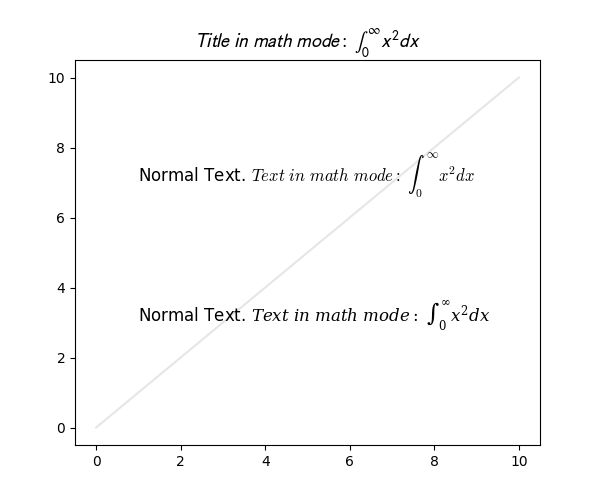# Math fontfamily#

A simple example showcasing the new math_fontfamily parameter that can be used to change the family of fonts for each individual text element in a plot.

If no parameter is set, the global value rcParams["mathtext.fontset"] (default: 'dejavusans') will be used.import matplotlib.pyplot as plt

fig, ax = plt.subplots(figsize=(6, 5))

# A simple plot for the background.
ax.plot(range(11), color="0.9")

# A text mixing normal text and math text.
msg = (r"Normal Text. $Text\ in\ math\ mode:\ " r"\int_{0}^{\infty } x^2 dx$")

# Set the text in the plot.
ax.text(1, 7, msg, size=12, math_fontfamily='cm')

# Set another font for the next text.
ax.text(1, 3, msg, size=12, math_fontfamily='dejavuserif')

# *math_fontfamily* can be used in most places where there is text,
# like in the title:
ax.set_title(r"$Title\ in\ math\ mode:\ \int_{0}^{\infty } x^2 dx$",
math_fontfamily='stixsans', size=14)

# Note that the normal text is not changed by *math_fontfamily*.
plt.show()


Keywords: matplotlib code example, codex, python plot, pyplot Gallery generated by Sphinx-Gallery